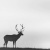IEEE模糊系统会刊TFS(IEEE Transactions on Fuzzy Systems)是由IEEE所属神经网络联合会发起和创办的一种新出版物。刊登有关模糊系统的理论、设计和应用方面的高质量技术论文,特别重视工程系统和科学应用,同时刊登信息以及有关其所载文章的评论和反驳。 官网地址：http://dblp.uni-trier.de/db/journals/tfs/0+0+0+0+0+0+0+0+0+0+0+0+0+0+0+0+0+0+0+0+0+0+0+0+0+0+0+0+0+0+0+0+0+0+0+0+0+0+0+0+0+0+0+0+0+
Top